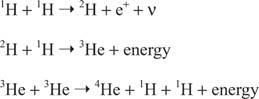## Energy Generation: Proton‐Proton Cycle

The energy radiated away from the solar photosphere is generated in the solar interior by thermonuclear reactions involving the fusion of four nuclei of hydrogen to one nucleus of helium. Temperatures are high enough for this to occur only in the central 25 percent of the Sun, called the core.

The relevant nuclear reactions are governed by only four physical principles: conservation of electrical charge (the net electrical charge does not change in a reaction); conservation of leptons (leptons are lightweight nuclear particles such as electrons e , positrons e +, and neutrinos ν); conservation of baryons (baryons are heavy nuclear particles such as protons and neutrons, also called nucleons); and conservation of mass‐energy (mass m and energy E are equivalent forms, related by Einstein's equation E = mc 2 where c is the speed of light). The specific process that occurs in the central region of the sun begins with the combination of two hydrogen nuclei or protons, hence is termed the proton‐proton cycle. To keep track of the various particles that make up the nuclei that are involved in these reactions, a notation such as mX may be used, where m represents the total number of particles (neutrons plus protons) in the nucleus, and X is the chemical species of the nucleus, equivalent to specifying the number of protons in the nucleus. 1H therefore is the normal form of hydrogen, which consists of a single proton; 2H is a heavier form of hydrogen, deuterium, which contains a neutron in addition to the proton; 4He the common form of helium with two protons and two neutrons; and so forth. The basic proton‐proton cycle is the sequence:For each kilogram of hydrogen, about 0.007 kg disappears into energy via E = mc 2 because helium has less mass than four individual hydrogen atoms. To account for the solar luminosity thus requires about 4 × 10 38 reactions each second; in other words, the conversion of approximately 6 × 10 11 kilograms of hydrogen to helium each second.

At the temperatures that prevail in the solar core, the Standard Solar Model predicts that about 8 percent of the time, the last step in the above sequence is replaced byand about 1 percent of the time, another alternative occursBack to Top
A18ACD436D5A3997E3DA2573E3FD792A